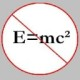You are currently browsing the tag archive for the ‘Propositional Calculus’ tag.

Let’s see if we can build this from ground up. We first define a statement (or sometimes, a proposition) to be a meaningful assertion that is either true or false. Well, meaningful means we should be able to say for sure if a statement is true or false. So, something like “Hello, there!” is not counted as a statement but “the sun is made of butter” is. The latter is evidently false but the former is neither true nor false. Now, it can get quite cumbersome after a while if we keep using statements such as “the sun is made of butter” every time we need to use them. Thus, it is useful to have variables, or to be precise, propositional variables, to denote all statements. We usually prefer to use$p, q, r$ and so on for such variables.

Now, all of this would be rather boring if we had just symbols such as$p, q, r$ etc. to denote statements. Thus, a statement like “Archimedes was a philosopher” is not that interesting in itself. In fact, all the statements (in our formal system) would be “isolated” ones in the sense that we wouldn’t be able to logically “connect” one statement to another. We want to be able to express sentences like “$x = -2$ and$y=2$“, “$(x = -2)$ implies$(x^2 = 4)$” and so on. So, we add something called logical connectives (also called operator symbols) to the picture. There are four basic ones:$\wedge$ (conjunction),$\vee$ (disjunction),$\rightarrow$ (material implication), which are all of arity 2 and$\neg$ (negation) which is of arity 1. Using these logical connectives, we can now form compound statements such as$p \wedge q$ (i.e.$p$ and$q$),$p \vee q$ (i.e.$p$ or$q$),$\neg p$ (i.e.$\mbox{not} (p)$), and$p \rightarrow q$ (i.e.$p$ implies$q$.) Note that each of$\wedge, \vee$ and$\rightarrow$ requires two propositional variables in order for it to make any sense; this is expressed by saying their arity is 2. On the other hand,$\neg$ has arity 1 since it is applied to exactly one propositional variable.

We also introduce another logical operator called logical equivalence ($\equiv$,) which has arity 2. It is really convenient to have logical equivalence on hand, as we shall see later. We say$p \equiv q$ if and only if “$(p \rightarrow q) \mbox{ and } (q \rightarrow p)$“. What this basically means is, if$p$ is true then so is$q$ and if$q$ is true then so is$p$. Another equivalent way of saying this is, if$p$ is true then so is$q$ and if$p$ is false then so is$q$.

Before we proceed further, we make a few observations. First, if$p$ and$q$ are propositional variables, then by definition each of those is either true or false. Formally speaking, the truth value of$p$ or$q$ is either true or false. This is equally true of the compound statements$p \wedge q,\, p \vee q,\, p \rightarrow q$ and$\neg p$. Of course, the truth values of these four compound statements depend on$p$ and$q$. We will delve into this in the next post.

Second, we don’t really need all the four basic operators. Two of those, viz.$\rightarrow$ and$\neg$ suffice for all logical purposes. This means all statements involving$\wedge$ and/or$\vee$ can be “converted” to ones that involve only$\rightarrow$ and$\neg$. However, we can also choose the “minimal” set$\{ \wedge, \,\neg \}$, instead, for the purpose for which we chose the minimal set$\{ \rightarrow, \neg \}$. In fact, there are lots of other possible combinations of operators that can serve our purpose equally well. Which minimal set of operators we choose depends sometimes on personal taste and at other times on practical considerations. So, for example, while designing circuits in the field of computer hardware, the minimal operator set that is used is$\{ \downarrow \}$. In fact, all that’s really needed is this particular operator set. Here$p \downarrow q \equiv \neg (p \wedge q)$.

So, what have we got so far? Well, we have a formal notion of a statement (or proposition.) We have access to propositional variables ($p, \, q, \, r$, etc.) that may be used to denote statements. We know how to create the negation of a given statement using the$\neg$ logical connective. We also know how to “connect” any two statements using conjunction, disjunction and material implication that are symbolically represented by the logical connectives$\wedge, \, \vee$ and$\rightarrow$, respectively. And, lastly, given any two statements$p$ and$q$, we have defined what it means for the two to be logically equivalent (which is symbolically represented by$\equiv$) to each other. Indeed,$p \equiv q$ if and only if ($p \rightarrow q \mbox{ and } q \to p$).

We shall see in the later posts that the above “small” formal system (for propositional calculus) we have built thus far is, in fact, quite powerful. We can, indeed, already employ quite a bit of it in “ordinary” mathematics. But, more on this, later!

I wish to use this part of the blog to quickly go through the basic elements of propositional calculus, and then later move on to predicate calculus in another part of the blog, followed by the fundamentals of relational algebra in yet another part. I might then go through the problem of query optimization in RDBMS after that. Let’s see how far this goes.

### Blog Stats

• 349,162 hitsprof dr drd horia or… on My first postprof drd horia orasa… on My first postprof dr mircea orasa… on Inequality with lognotedscholar on Self-referential Paradoxes, In…prof dr mircea orasa… on Inequality with logprof dr mircea orasa… on Inequality with logprof dr mircea orasa… on 2010 in reviewkenji on Basic category theory, Iprof dr mircea orasa… on Solution to POW-10: Another ha…prof drd horia orasa… on Continued fraction for eprof drd horia orasa… on Inequality with logprof dr mircea orasa… on Solution to POW-12: A graph co…prof dr mircea orasa… on The Character of Physical…prof dr mircea orasa… on Milk and Tea puzzleprof drd horia orasa… on Inequality with log